# Python For Loop

A loop structure in programming language is to repeat a piece of code or block several times. Python has several types of loops such as for loop, for-each loop, and while loop.

In this article, you will learn about for loop structure. A for loop is used when you know exact number of times a loop is going to run. Each time a code is executed within the loop is called an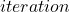.

Suppose you want to count how many item in a shopping list. Then you go through each item of the list is an example of loop. Here you are looping through each item of the list.

### Python For Loop Structure

The traditional way of writing a for loop in python is as follows.

# for loop structure
for i in range([start], end, [step]):
do something
do something

Let’s understand the above code in detail. The loop starts with a keyword called for which is a reserved keyword in python. The a variablerepresents each item in a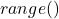or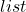. This variabletake one value during each iteration from the range. The range function represents a list of numbers that has a start and ending number. The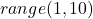will include all numbers starting fromto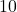. However, it includes only starting number and does not include the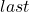or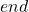number.

The step is optional, and it tells the loop to skip few numbers or loop in steps of some value. Consider the following example.

# This example prints all number in a range.
for i in range(1,10):
print(i)

The above for loop will assign a number fromtoto the variableduring each iteration. Then the value ofis printed as output. The output of the program is given below.

1
2
3
4
5
6
7
8
9

Note that the loop does not print the last digit in the range.

### Different Types of Ranges

You can set the range function in different ways. Here are some examples.

Range without start

# This is example of for loop with range without a start
for i in range(5):
print(i)

The loop will print all number starting from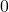to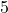with except the last digit.

0
1
2
4

Range with steps

# This example prints all the even numbers
for i in range(0, 10, 2):
print(i)

This loop will print all the even numbers betweentoexcept. The number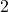in the range allow the loop to print the second digit from the current value of. Suppose ifstarts with, then the next value ofwill beand so on.

0
2
4
6
8

Range in reverse order

Sometimes you want to loop through in reverse order, that is, the higher number is first and lowest number is last. Again, the last digit is skipped. Consider the following example.

for i in range(10,0, -1)
print(i)

The above range is printed in reverse order.

10
9
8
7
6
5
4
3
2
1

Note that the last digit 0 is not printed. In the next article, you will learn another way of writing the for loop.